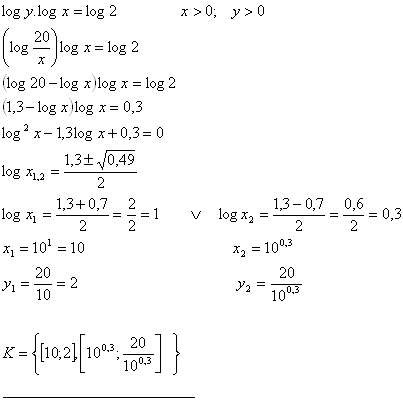sk|cz|

# Sets of Logarithmic Equations

1. Solve the set of equations:

x + y = 34
log2x + log2y = 6        x,y >0 , x < 34
Solution:
x + y = 34
log2x + log2y = 6        x,y >0 , x < 34

y = 34 -x

log2x + log2(34 – x) = 6
log2 ( x.(34 – x)) = log264
x.(34 –x)  = 64
x2 – 34x + 64 = 0
(x- 32)( x-2) = 0
x1 = 32 v x2 = 2

y = 34 – x
y1 = 34 – 32 v y2 = 34 - 2
y1 = 2 v y2 = 32

K = {[32,2][2,32]}

2.Solve the set of equations: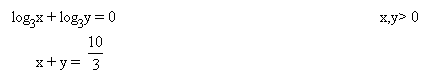Solution: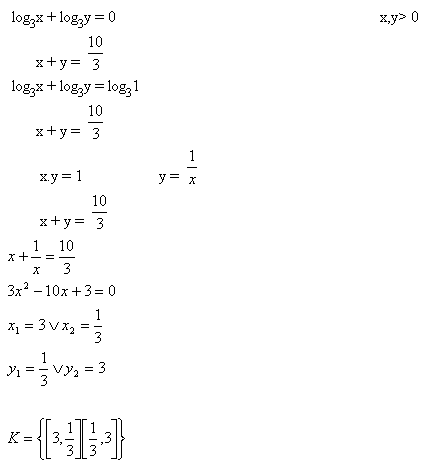3.Solve the set of equations: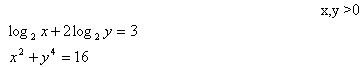Solution: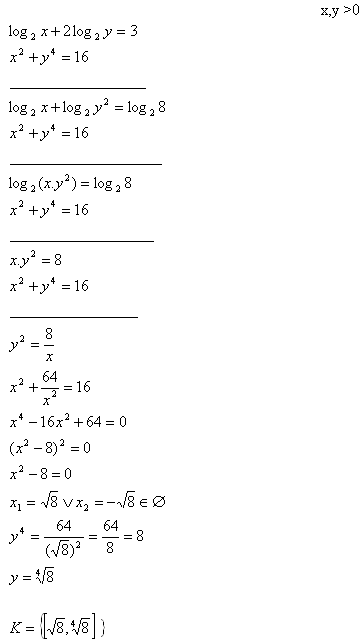4.Solve the set of equations: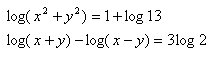x>0, y>0, x>y
Solution: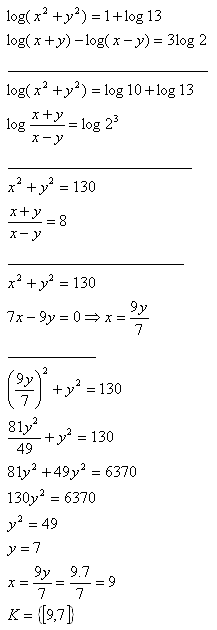5.Solve the set of equations: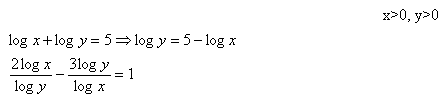Solution: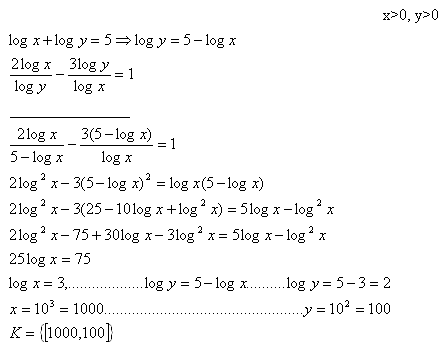6.Solve the set of equations: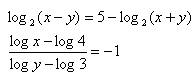x>0, y>0
Solution: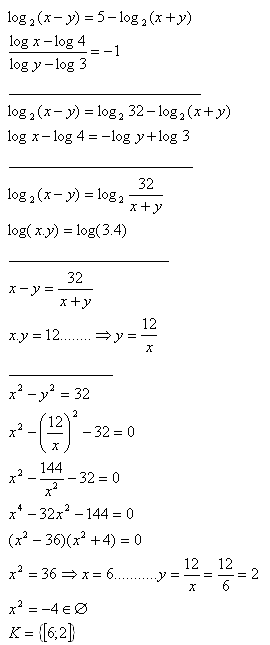7.Solve the set of equations: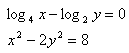x>0, y>0
Solution: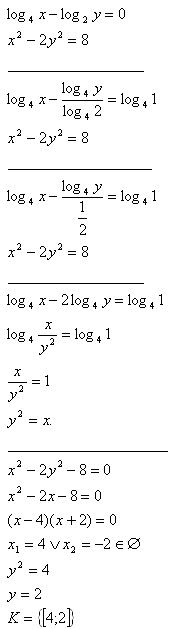8.Solve the set of equations: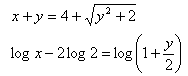x > 0; y > 0
Solution: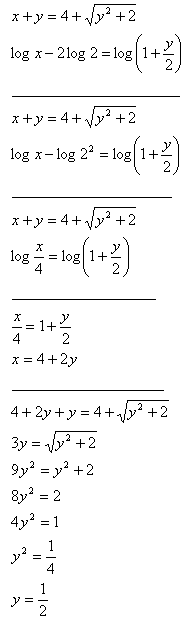x = 4 + 2y
x = 4 + 2.0,5
x = 4 + 1
x = 5

K = {5;1/2}

9.Solve the set of equations: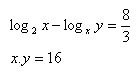Solution: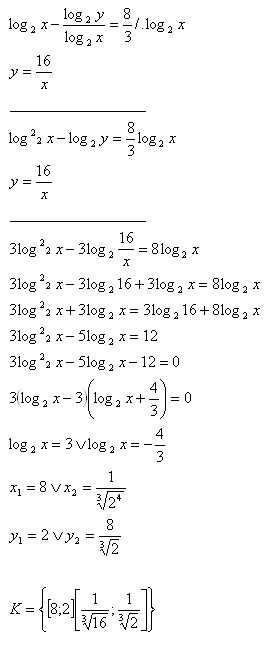10.Solve the set of equations: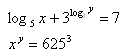x > 0 ; y> 0
Use the formula: 3log3y = y
Solution: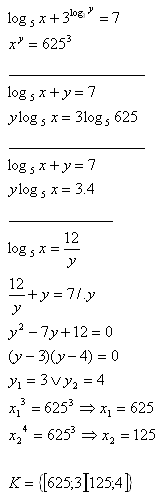11.Solve the set of equations: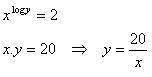Solution: